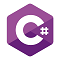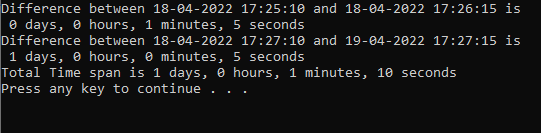# Blog# Find the difference between DateTime Fields in C#

623  24-April-2022

In this blog, we will learn How to find the difference between two DateTime fields in C#.In this blog, we will learn How to find the difference between two DateTime fields in C#. For demonstration, I will take two DateTime variables and will find the difference between them. TimeSpan object represents the difference between the two DateTime.

TimeSpan Struct has properties like Days (to get the days component of the time interval represented by the current TimeSpan structure), Hours (to get the hours component of the time interval represented by the current TimeSpan structure), Minutes (to get the milliseconds component of the time interval represented by the current TimeSpan structure), Seconds (to get the seconds component of the time interval represented by the current TimeSpan structure), etc.  TimeStamp Struct has several methods out of which I am using Add(TimeStamp) which returns a new TimeSpan object whose value is the sum of the specified TimeSpan object and this instance.

``````//Take two DateTime fields
DateTime dateTimeFrom1 = DateTime.Parse("18-04-2022 17:25:10");
DateTime dateTimeTo1 = DateTime.Parse("18-04-2022 17:26:15");

//Difference in From and To DateTime is 1 minute and 5 sec
TimeSpan timespan1 = dateTimeTo1.Subtract(dateTimeFrom1);

Console.WriteLine(string.Format("Difference between {0} and {1} is \n {2} days, {3} hours, {4} minutes, {5} seconds",
dateTimeFrom1, dateTimeTo1, timespan1.Days, timespan1.Hours, timespan1.Minutes, timespan1.Seconds));

//Let's again find difference between two dates
DateTime dateTimeFrom2 = DateTime.Parse("18-04-2022 17:27:10");
DateTime dateTimeTo2 = DateTime.Parse("19-04-2022 17:27:15");

//Difference in In and Out Time is 1 Day and 5 sec
TimeSpan timespan2 = dateTimeTo2.Subtract(dateTimeFrom2);

Console.WriteLine(string.Format("Difference between {0} and {1} is \n {2} days, {3} hours, {4} minutes, {5} seconds",
dateTimeFrom2, dateTimeTo2, timespan2.Days, timespan2.Hours, timespan2.Minutes, timespan2.Seconds));